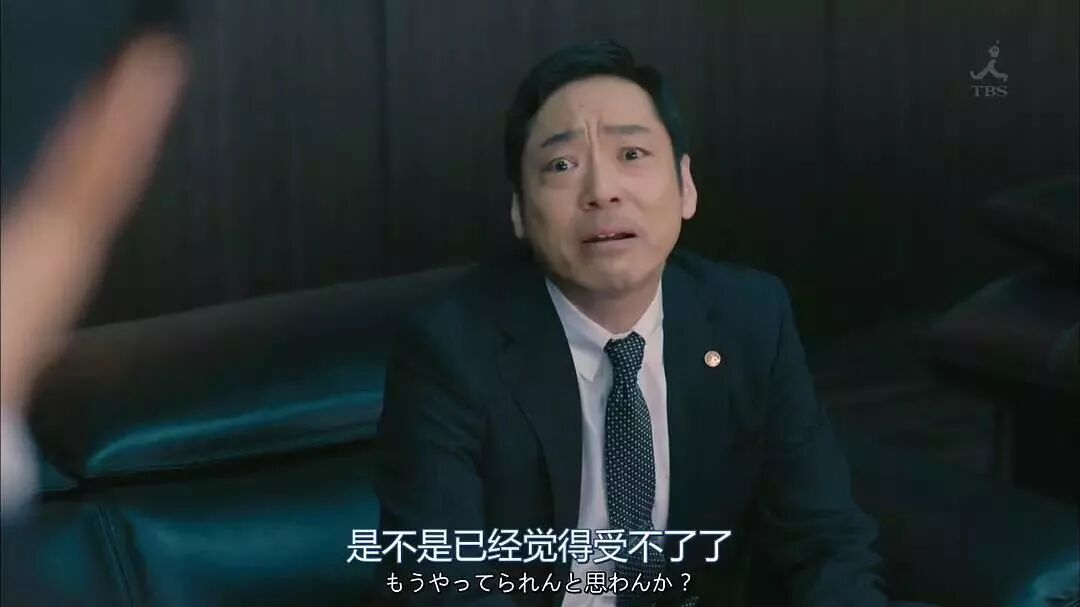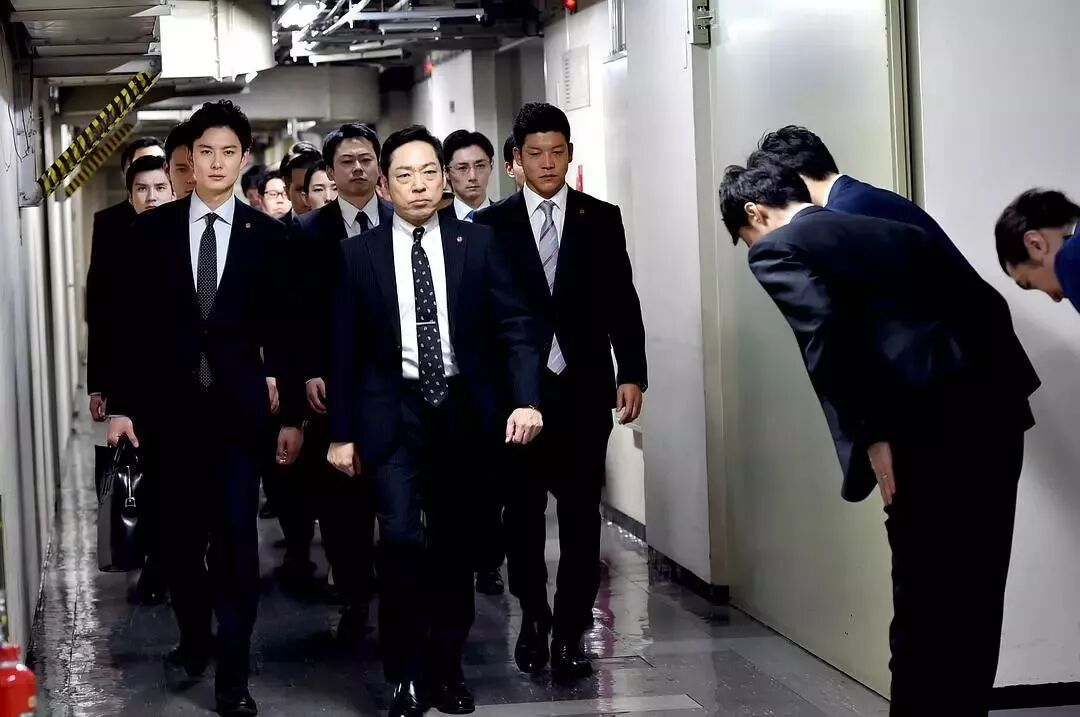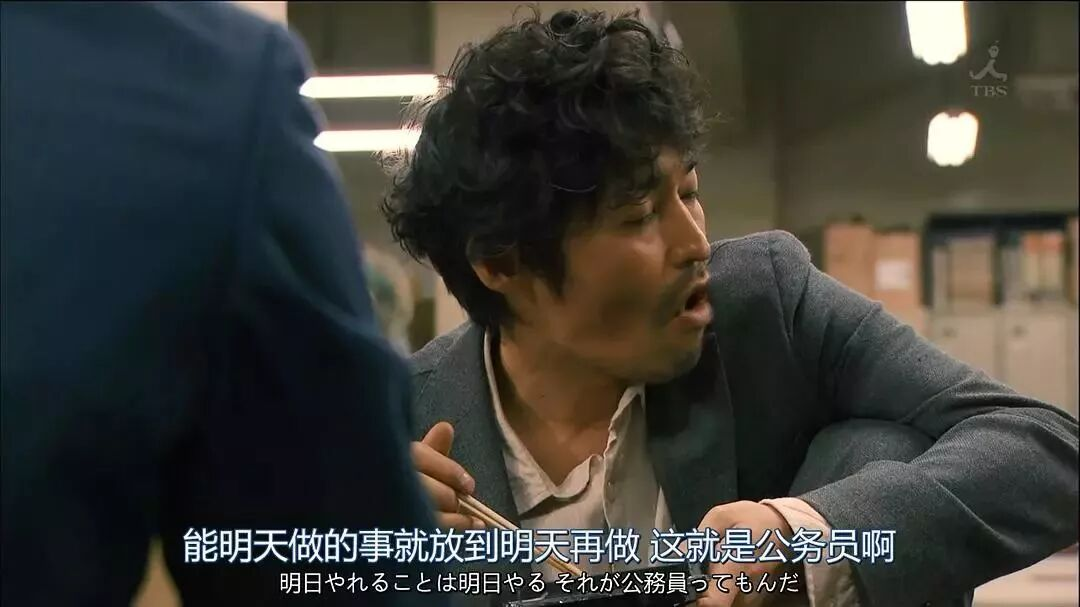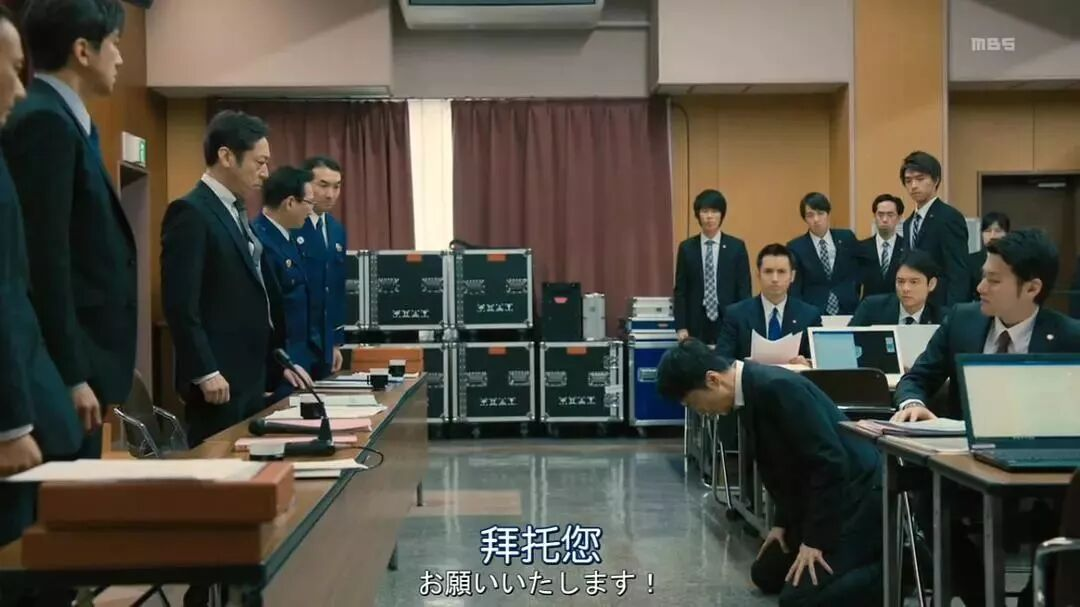## [同事亲友] 那些离开体制内的年轻人||真实故事计划 麦子熟了

### 马上注册，结交更多好友，享用更多功能，让你轻松玩转社区。

x
0 ^  R# ?9 c4 U! Z, i) b+ ]( `4 G\$ J& h

( r: ^& s4 |' A# U* I
" X! ^) J& c0 w5 H+ @- q

- o4 z* i. }6 T: z1 p

1 h\$ U/ W% i7 g+ b, T5 Y; I

\$ ~9 T, ]- N1 n4 {

3 a& s5 ]3 g8 L2 @

! v; |4 T) v3 l4 A

- `, U3 d6 m" |9 t) ]( C. W) ?- M
1月25日，国考笔试出分。又有一批年轻人即将进入体制内，拿起铁饭碗。

5 f& I" F' v# c1 `7 y
; I1 t9 l4 [: q: h' [8 [3 M  m& W
) L+ J# T0 ?1 r1 t  d3 Z1 J0 C

. ?, Y, z/ c  v' U. Q. V9 K

8 I; s; K8 x, |( j
3 L% a0 f# ~- N4 X" _  F
8 m- m+ o/ j/ E' d; _: L

Part
1

2 F8 _' e' b, L0 |+ X

& j2 F2 r+ h3 j
# k7 {. b5 T  t1 X

2 T0 z4 ~: ?\$ J2 ~& Z1 c6 l! ^7 r
+ b2 ]% h# B1 |! o' k

\$ `# W7 b% k4 D! Q  y\$ x: r

0 X/ N, a! s! f* A8 ]
“跳车！跳啊！快他妈跳啊！”
9 d5 ]) S4 r" S' e. U2 W

/ V# F* Z& W! i+ }1 ^- o3 i

/ S+ X6 J+ f4 _6 S. @8 V& _) Y. y. }

3 ?& z/ P, E1 k! j
1 D, f  M* S; R8 Q- \% z9 i\$ i4 F3 {" M/ q  O: }) o9 X
) f; c9 w/ `) w  _6 k# x; G

6 B* _9 \: C* h3 L% J

9 O  V( s+ w3 p2 n9 {

/ S8 N6 H: `1 J+ h3 t

: f# L8 _" l# C4 u3 I

" e7 H7 L/ C. o& q6 m

1 C7 n  M/ D\$ h) h4 Q; O& \

9 `, B# ^) o/ P0 q) G

, l  z9 |/ D2 K* ]7 |/ p2 n1 V9 T
7 ~: h; Y3 G( m' E# `, w+ [/ L% V% I5 [) b9 r4 H+ T/ x* Q) A

7 c5 @/ \- m  m6 P/ j) c9 ?9 y: ]

# W, z& W& E& \) {

- {. m6 g\$ g* b2 V\$ n: ?* Q0 J

8 ]5 E1 |4 _4 R1 Z- o: B- a& W, o

+ r2 F; j+ o* }6 v% ~\$ x& ]' L3 z" F; ^& W9 p

/ k2 W\$ R! p. ]& g- i# w! k7 a

; O+ }/ L3 Q+ O

4 D+ W0 [+ J+ `# C( B* ^1 b" K4 p! M! N4 q9 O
" c0 {% s7 Z2 S. ?: i\$ \+ W& L
; z% F\$ Q) |: Y/ n0 C' f

/ ^2 L2 ~2 [% E8 ?

Part
2

/ H- b5 S+ Z' n: g9 y

5 s3 m0 v" v9 \0 [* c6 u

2010年，我拿到了大专自考本科的文凭，当时伯伯是烟草局里的二把手，给弄了个名额，我就开始在市里的烟草局上班。
4 O) e: j% P! H  C

) p  b6 T" K1 M/ R4 W+ q, L( a; Q

, ^/ m8 z2 b5 H: A& M4 w

“我刚从后面看，还以为哪个大叔走错包间了。”
: a% l; u8 n: t\$ d9 E; ]( Q

. Z  `% u' ~  y\$ i7 i! `" B/ O+ Z) w8 @; S' {' Q- |- o

8 p% z/ K( ?+ C& f9 n
: h! m8 \/ [" q( U( X5 `

7 x( {+ p, R9 X& R7 q+ k

( q\$ @  T& V# ~- o0 w  W/ v
“以前都是我错了，我余生给你做牛做马，你不要糟蹋自己。”

' Y3 j9 Q- w# `; Y! N* W

' t1 o5 I4 w1 q; ?3 |3 J1 T( G7 a- k( Y( P\$ y6 \2 D# a' |
; p3 h' A. B" ~( f7 \+ ]  U/ Q! t

. |7 J2 f' H* E" t" V5 Q

; V1 D! H' ^& x# _

1 O% H3 {. x7 p\$ M7 s5 z% X
“冤枉了就冤枉了，老子就是要冤枉他。”

: Y& f0 A: T" b

: K\$ T- W' e+ e

+ R0 G5 f* l+ x9 C# z- P7 ]

# o3 E. B) E. N6 F) }

" T9 C\$ S, Z8 O, _7 r) }- G% R3 c

* r0 X- g3 x# p9 ?

R7 L# ]2 n" p' O' d' x& p

1 j1 {% U+ E# k* b\$ j* p7 q

( T\$ v% j, Z& Y% n: y5 y' i, e

6 P8 P\$ e* x& g, G5 B: V( O  h, M! Q6 G  M7 y# g# l

, M% P/ R& ], m# F0 v4 _

6 a! ~5 Z8 `/ M7 a& e" s8 P; n8 J

' C# r# k: R& d! p8 J/ V: X
: j1 x- |: ?; j5 P2 X7 k7 Z9 P! w3 S  k+ G8 i7 o
! D3 Z; Z( ~\$ {6 e/ E4 \; ^

- l" H- u; ]% k! F; U+ Q2 b! u

5 ^# J\$ u/ K& x3 S- z1 Y! g! }
( u5 H\$ P/ u7 b; u

( O2 O6 ^) x0 S' d5 A
4 }& [. a. _- _  U+ U* l0 r

" [7 l1 t* S& _- T! r; b% w! q( w) i2 m- {  f# p1 x8 C

4 e9 K* D4 T. Z\$ Z
8 F6 S) u2 F\$ C/ J9 K

) x: a7 G# f" }0 K1 C8 Y& G' e

3 V5 b4 Y, T  N' f* l% R' g

0 ]* ^, M1 v# k. R& L# e0 s
* s& s, x5 r: x  b

" o' t: G8 H5 j" d2 z. N
; M6 H7 q1 C! M- J: o1 k. a

\$ n# `/ d+ E9 S) `0 j3 ^2 u2 o

+ [, Q- m* W0 b% ?! Y# @6 q
2 G7 j: j0 z. E8 a

4 b* @/ _4 J! n! J6 N5 H5 e

( X. k& I5 `9 y( ~" ?, d, {

! q4 L* y6 m; b3 ?8 l  n

\$ x: l  |1 r0 i% }& @, U- p

2017年，从年初十到五一，他每天早上七点出门，晚上十二点回家，没有机会说话。
5 \  ^! U) q# }4 Q

# e) o4 ?% u9 J8 O- I

\$ B* Q\$ w' s" M% M5 t% N! h
P0 _' J5 \+ t- ~4 X1 w- E! z. g4 J

3 G; ?  L" K# S% ^

( Q9 h. n7 I! r. D* M/ O' X4 @

' K, o0 c2 j6 f

) W6 c6 T/ L% }, q

/ {' x& h" f( I3 k

1 z# v3 X: G: m: H; K6 l

+ c( n\$ ?3 H% S1 M

“我们是副股级后备干部。”
5 J0 \' q4 t8 b

( ]3 W8 G5 N- ^" B7 C: N) }+ r2 H2 N6 J6 w) a. R% m
8 h/ V7 I# j1 Y
2014年中期，媒体行业的不景气已经扩散开来，很多同事都选择了辞职，加入大的互联网公司，甚至是自己创业。

. X' k\$ n7 ?6 _  B6 ?

4 G" [. p% a0 y4 @5 H0 ?

' F" |( I: A1 @9 H. X* h+ j

! q& ]4 b/ G9 Z+ |

' V& Q/ Z" e+ y4 E" u( M

: v7 x9 j7 k- c  d! Z# I

1 q& ~9 R, V/ m+ i+ b  W/ `8 j

) e) [4 Q0 K% M' `\$ d0 i, C- }( B1 F7 g7 i& \8 l) E, F

k1 ~" `2 V- n" R\$ @  o( A
“我们只能谈这些很俗的事情了。”
" F  g( |8 x2 V* ^0 P3 c
% k/ R" [; ]\$ s3 X/ h

" z3 N% q8 G\$ L2 @# D7 ?" [1 G

1 [4 A4 k) x% O# X1 j2 U% E

6 V8 j, j1 A8 v' V# S

( c3 q2 ?3 g\$ I( `, f- W

; L6 |) |: g) M% n7 h
: x) I% e6 Z4 `- S
Part
3
: k0 |- ]- M! V9 l# N2 n5 s4 D

s9 `" Y+ l! [6 o; G

2 G" K\$ t6 y6 E" I* T* t
8 {* i4 ~( K; \6 `6 W: P- a

~0 L. d. S\$ `  ^

% W! F% Q) H\$ ]4 j! t
* G0 ^8 X\$ w\$ P

( x+ f+ H, U: r# [# `# P
4 q( {, f0 q" S0 |0 k% G3 s! b* s. m9 Q! Z) |5 ^

4 F; J4 g( y# O: j

8 }9 ]  b6 T1 I6 ?' [' j. R, m
& z  _. v6 x6 z, ~2 j8 }

1 b* M- I3 h( h# M4 H

. X0 i\$ X2 k6 c; T8 u5 o. J

c# ?* ]\$ G* Y2 {/ h

- \+ Q: `\$ C\$ K) j

, E: _' X7 \8 N+ v* V8 b

3 f7 T8 N& c  h" y9 o6 H# S3 l- _# |* P" [( P5 c% m* U

/ d\$ H( }7 C; q3 M  m

“你滚吧。”
, Z# v3 d2 t" ?" m% f) r“领导，我滚出去了，现在可以让我坐下了吧？”

“那你先坐那儿吃饭吧。”
+ H/ t- q0 G/ X# g9 b2 N

+ w# k3 i7 \8 w/ b/ b' n3 C

0 s1 P& d3 S; Z

9 h/ n5 S: ]7 M) q+ T, b
( Z% t4 D& F/ j4 ]  p/ y

" V2 v' r6 K\$ W2 s: G

! M" T1 h+ B' ~1 r6 h- E* ~

*作者：真实故事计划，公众号：真实故事计划（ID: zhenshigushi1）。真实故事计划是由青年媒体人打造的国内首个真实故事平台，这里每天讲述一个从生命里拿出来的故事。

— END —## 使用高级回帖 (可批量传图、插入视频等)快速回复您需要登录后才可以回帖 登录 | 注册 本版积分规则   Ctrl + Enter 快速发布   回帖后跳转到最后一页 发帖时请遵守我国法律，网站会将有关你发帖内容、时间以及发帖IP地址等记录保留，只要接到合法请求，即会将信息提供给有关政府机构。